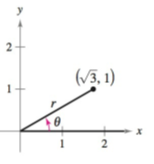Chapter 8.2, Problem 1CP### Calculus: An Applied Approach (Min...

10th Edition
Ron Larson
ISBN: 9781305860919

#### Solutions

Chapter
Section### Calculus: An Applied Approach (Min...

10th Edition
Ron Larson
ISBN: 9781305860919
Textbook Problem
1 views

# Let ( 3 ,   1 ) be a point on the terminal side of θ, as shown in the figure. Find the sine, cosine, and tangent of θ.To determine

To calculate: The value of the sine, cosine, and tangent for the provided figure if (3,1) be the point on the terminal side.

Explanation

Given Information:

The value of x is 3 and y is 1 and the provided figure is shown below,

Formula used:

Circular function definition:

sinθ=yr         cscθ=rycosθ=xr         secθ=rxtanθ=yx         cotθ=xy

Where, x is the length of the side adjacent to θ, y is the length of the side opposite to θ, and r=x2+y2 is the length of hypotenuse which is not equal to 0.

Calculation:

From the provided figure, the value of x is 3 and y is 1. So, the value of r will be:

r=x2+y2=(3)2+12=3+1=4

On further simplification,

4=2

Thus, r=2

### Still sussing out bartleby?

Check out a sample textbook solution.

See a sample solution

#### The Solution to Your Study Problems

Bartleby provides explanations to thousands of textbook problems written by our experts, many with advanced degrees!

Get Started

#### Rationalize Numerator Rationalize the numerator. 91. 153

Precalculus: Mathematics for Calculus (Standalone Book)

#### Solve the equations in Exercises 126. 14x2=0

Finite Mathematics and Applied Calculus (MindTap Course List)

#### In Problems 35-42, simplify each complex fraction. 40.

Mathematical Applications for the Management, Life, and Social Sciences

#### For y = sin 6x, dydx=. a) cos 6x b) 6 cos x c) cos 6 d) 6 cos 6x

Study Guide for Stewart's Single Variable Calculus: Early Transcendentals, 8th